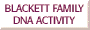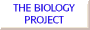## Blackett Family DNA Activity 2

### Genotype Probability at any STR Locus

• Part of the work of forensic DNA analysis is the creation of population databases for the STR loci studied.
• Probability calculations are based on knowing allele frequencies for each STR locus for a representative human population (and showning Hardy-Weinberg equilibrium for the population by statistical tests).
• Allele frequency is defined as the number of copies of the allele in a population divided by the sum of all alleles in a population.
• For a heterozygous individual, if the two alleles have frequencies of p and q in a population, the probability (P) of an individual of having both alleles at a single locus is

P = 2pq

• If an individual is homozygous for an allele with a frequency of p, the probability (P) of the genotype is

P = p2.

• We saw earlier that Bob Blackett has the genotype 15, 18 at the locus D3S1358. In a reference database of 200 U.S. Caucasians, the frequency of the alleles 15 and 18 was 0.2825 and 0.1450, respectively. The frequency of the 15, 18 genotype is therefore

P = 2 (0.2825) (0.1450) = .0819, or 8.2%.

### Probability for a DNA profile of Multiple Loci

• If databases of allele frequency for different loci can be shown to be independently inherited by appropriate statistical tests, the probability for the combined genotype can be determined by the multiplication (product rule).
• The probability (P) for a DNA profile is the product of the probability (P1, P2, ... Pn) for each individual locus, i.e.

Profile Probability = (P1) (P2) ... (Pn)

• The probability can be an extremely low numbers when all 13 CODIS STR markers are included in the DNA profile. As mentioned earlier, Bob Blackett calculated his own profile probability at 1.3 times 10-16, or no more frequent than 1 in 7.7 quadrillion individuals (7.7 million billion), which is more than a million times the population of the planet.

Introduction: Overview | STR P | CODIS | Analysis | Inheritance | Frequency Calc.

Activities : Pedigree | Collect data | Paternity testing | Missing person | RCMP freq. calc.The Biology Project
University of Arizona
October 27, 2000
hallick@email.arizona.edu
http://www.biology.arizona.edu